﻿ 柔体动力学模型的机器人柔性力矩前馈控制
«上一篇文章快速检索 高级检索

 哈尔滨工程大学学报2019, Vol. 40Issue (8): 1509-1516  DOI: 10.11990/jheu.2018070010

### 引用本文ZHANG Tie, ZHANG Aimin, QIN Binbin, et al. Flexible torque feed-forward control of robots in the flexible dynamics model[J]. Journal of Harbin Engineering University, 2019, 40(8), 1509-1516. DOI: 10.11990/jheu.201807001.### 文章历史

1. 华南理工大学 机械与汽车工程学院, 广东 广州 510641;
2. 桂林航天工业学院 广西高校机器人与焊接重点实验室, 广西 桂林 541004

Flexible torque feed-forward control of robots in the flexible dynamics model
ZHANG Tie 1, ZHANG Aimin 1, QIN Binbin 1, LIU Xiaogang 2
1. School of Mechanical and Automotive Engineering, South China University of Technology, Guangzhou 510641, China;
2. Guangxi Key Laboratory of Robotics and Welding, Guilin University of Aerospace Technology, Guilin 541004, China
Abstract: In this work, we aimed to solve the problem of robot's dynamic resonance hysteresis caused by complex structure, joint coupling, and nonlinear time-varying control and the problem of mechanical resonance caused by flexible factors. We achieve this objective by proposing a method for improving the compensation of rigid body feed-forward torque and for the feed-forward compensation control of flexible torque based on the flexible body dynamics model. Our method establishes the dynamics model of robot flexible joints and identifies the torsional stiffness parameters of flexible joints and the minimum inertia parameters necessary to obtain the location, speed, and acceleration information of the preset track under flexible factors with high accuracy and thus enable the calculation of the required torque value under the condition of flexible joints. The calculated values are considered as the feed-forward quantity and are sent in the form of the T cycle to the bottom of the servo driver. The values refresh the driver in real time and are superimposed in the form of compensation with the current loop output to enable flexible robot control. The amplitude of vibration acceleration at the end of the robot is reduced by 60%. Positioning accuracy is also improved. We perform an experiment to verify the value of the flexible torque feed-forward control method based on the flexible body dynamics model in engineering applications.
Keywords: robot    flexible body dynamics    stiffness coefficient identification    flexible position    feed-forward compensation

1 动力学建模 1.1 柔性关节动力学模型确定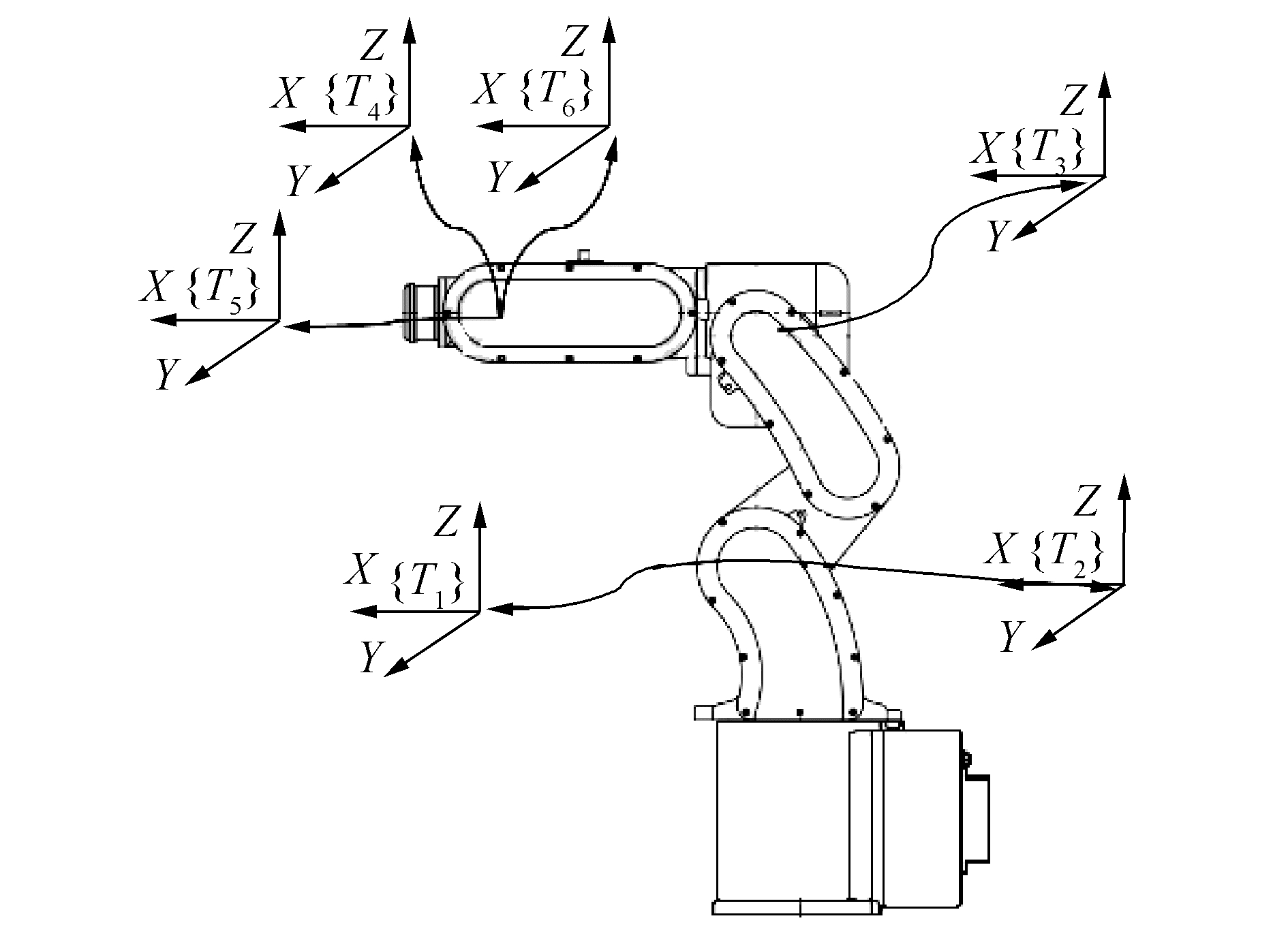Download: 图 1 垂直六自由度工业机器人 Fig. 1 Vertical six degree of freedom industrial robot

 $\tau_{i}=\sum\limits_{j=1}^{6} D_{i j} \ddot{q}_{j}+I_{a i} \ddot{q}_{i}+\sum\limits_{j=1}^{6} \sum\limits_{k=1}^{6} D_{i j k} \dot{q}_{j} \dot{q}_{k}+G_{i}$ (1)

1.2 标准辨识方程式建立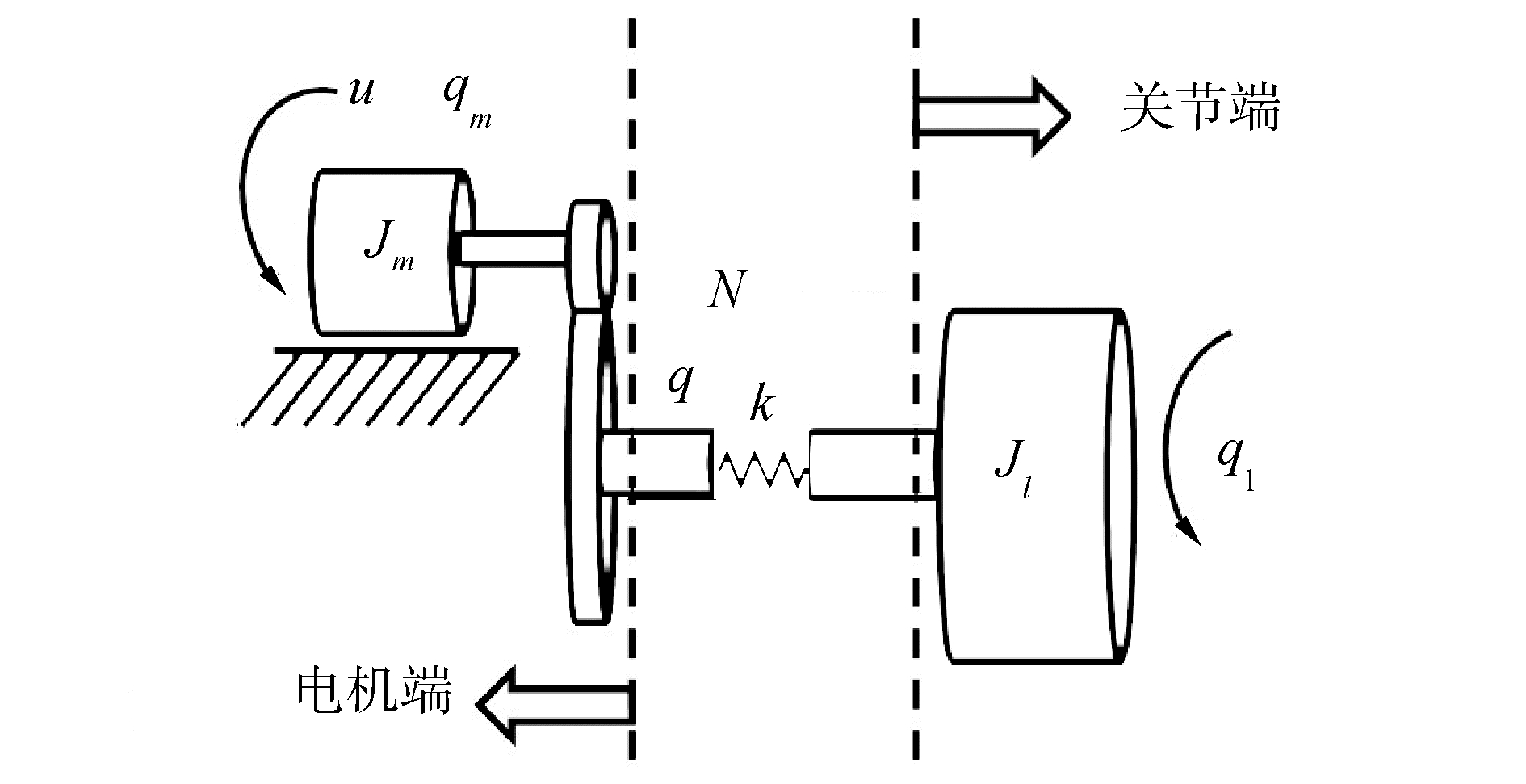Download: 图 2 电机-弹簧-质量的两惯量系统 Fig. 2 Two inertia system of motor-spring-mass

 $J_{1} \ddot{q}_{1}+f_{v j}\left(\dot{q}_{1}\right)+f_{s j} \operatorname{sign}\left(\dot{q}_{1}\right)+G\left(q_{1}\right)-k\left(q-q_{1}\right)=0$ (3)
 ${J_m}{\ddot q_m} + {f_{vm}}\left( {{{\dot q}_m}} \right) + {f_{sm}}{\mathop{\rm sign}\nolimits} \left( {{{\dot q}_m}} \right) + k\left( {q - {q_1}} \right) = {\tau _m}$ (4)
 $G\left(q_{1}\right)=M g X_{1} \cos q_{1}+M g Y_{1} \sin q_{1}$ (5)

 $\boldsymbol{\tau}=\boldsymbol{D} \boldsymbol{X}$ (6)

 $\mathit{\boldsymbol{\tau }} = {\left[ {\begin{array}{*{20}{c}} 0&{{T_{\rm{m}}}} \end{array}} \right]^{\rm{T}}},\mathit{\boldsymbol{X}} = \left[ {\begin{array}{*{20}{c}} {{J_1}}&{{J_{vj}}}&{{f_{sj}}}&{M{X_1}}&{M{Y_1}}&k&{{J_m}}&{{f_{vm}}}&{{f_{sm}}} \end{array}} \right]$
 $\mathit{\boldsymbol{D}} = \left[ {\begin{array}{*{20}{c}} {{{\ddot q}_1}}&{{{\dot q}_1}}&{{\rm{sign}}\left( {{{\dot q}_1}} \right)}&{g\cos {q_1}}&{g\sin {q_1}}&{ - \left( {{q_1} - q} \right)}&0&0&0\\ 0&0&0&0&0&{\left( {q - {q_1}} \right)}&{{{\ddot q}_m}}&{{{\dot q}_m}}&{{\rm{sign}}\left( {{{\dot q}_m}} \right)} \end{array}} \right]$
1.3 最小惯性参数模型

 ${q_1} = \left( {{J_m}{{\ddot q}_m} + {f_{vm}}\left( {{{\dot q}_m}} \right) + {f_{sm}}{\mathop{\rm sign}\nolimits} \left( {{{\dot q}_m}} \right) + k{g_m}/N - {\tau _m}} \right)/k$ (7)

 ${\dot q_1} = \left( {{J_m}{{\ddot q}_m} + {f_{vm}}\left( {{{\ddot q}_m}} \right) + {k_m}/N - {{\dot \tau }_m}} \right)/k$ (8)
 ${\ddot q_1} = \left( {{J_m}q_m^{(4)} + {f_{vm}}\left( {{{\ddot q}_m}} \right) + {{\ddot k}_m}/N - {{\ddot \tau }_m}} \right)/k$ (9)

 $\tau_{m}=\boldsymbol{W}_{s} \boldsymbol{X}_{s}$ (10)

 ${{\boldsymbol{W}}_s} = \left[ {\begin{array}{*{20}{c}} { - {{\ddot \tau }_m}}&{ - {{\dot \tau }_m}}&{q_m^{(4)}}&{{{\dddot q}_m}}&{{{\ddot q}_m}}&{{{\dot q}_m}}&{\operatorname{sign} \left( {{{\dot q}_m}} \right)} \end{array}} \right]$
 $\begin{array}{*{20}{c}} {{\mathit{\boldsymbol{X}}_s} = }\\ {\left[ {\frac{{{J_1}}}{k}\frac{{{f_{vj}}}}{k}\frac{{{J_1}{J_m}}}{k}\frac{{{J_1}{f_{vm}} + {J_m}{f_{vj}}}}{k}\frac{{k{J_m} + {f_{vj}}{f_{vm}} + {J_1}k/N}}{k}{f_{vm}} + \frac{{{f_{vj}}}}{N}{f_{sm}}} \right]} \end{array}$
1.4 动力学参数辨识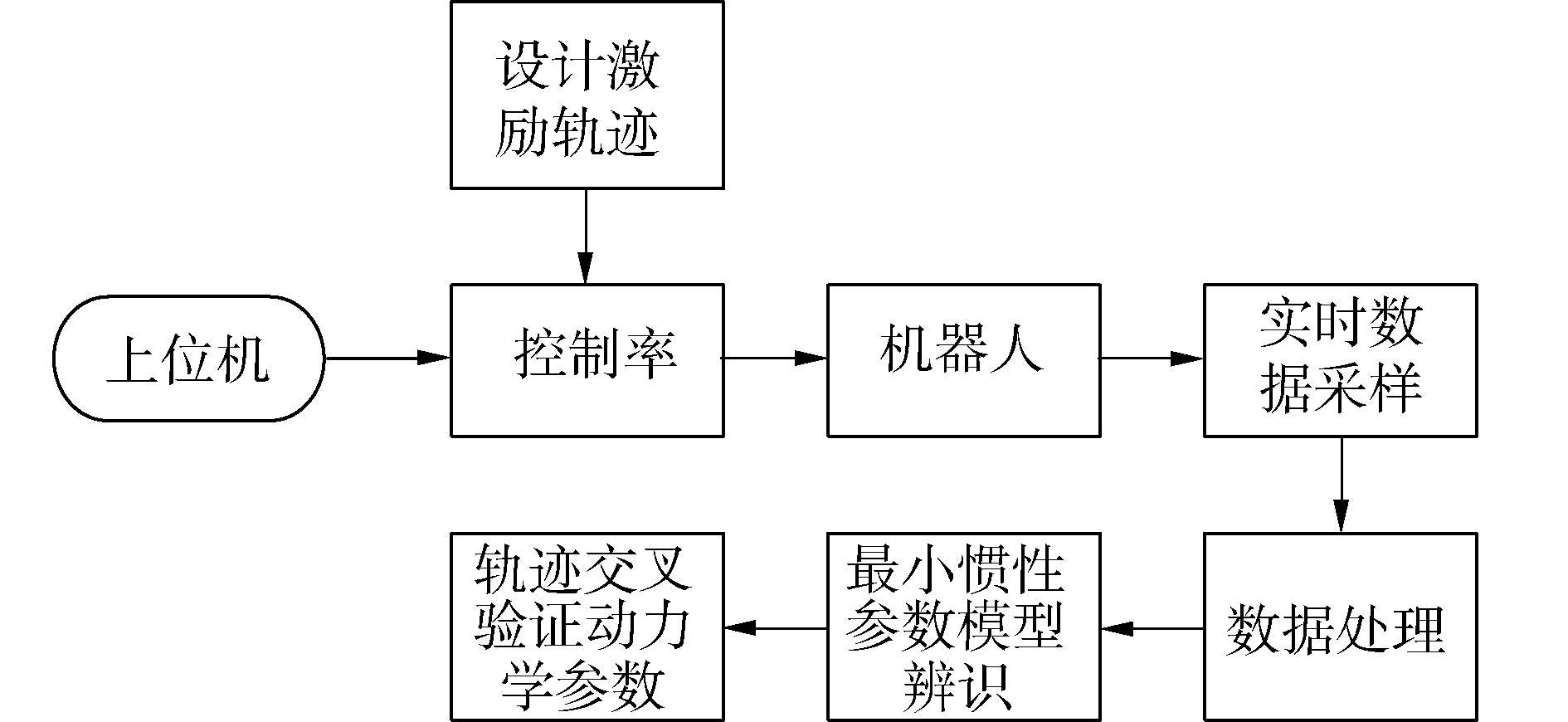Download: 图 3 参数辨识流程 Fig. 3 Flow chart of parameter identification

 $\dot q\left( k \right) = \frac{{q\left( {k + 1} \right) - q\left( {k - 1} \right)}}{{2{T_s}}}$ (11)
 $\ddot q\left( k \right) = \frac{{\dot q\left( {k + 1} \right) - \dot q\left( {k - 1} \right)}}{{2{T_s}}}$ (12)

 ${\omega _{{\rm{cutoff}}}} > 10{\omega _{{\rm{dyn}}}}$ (13)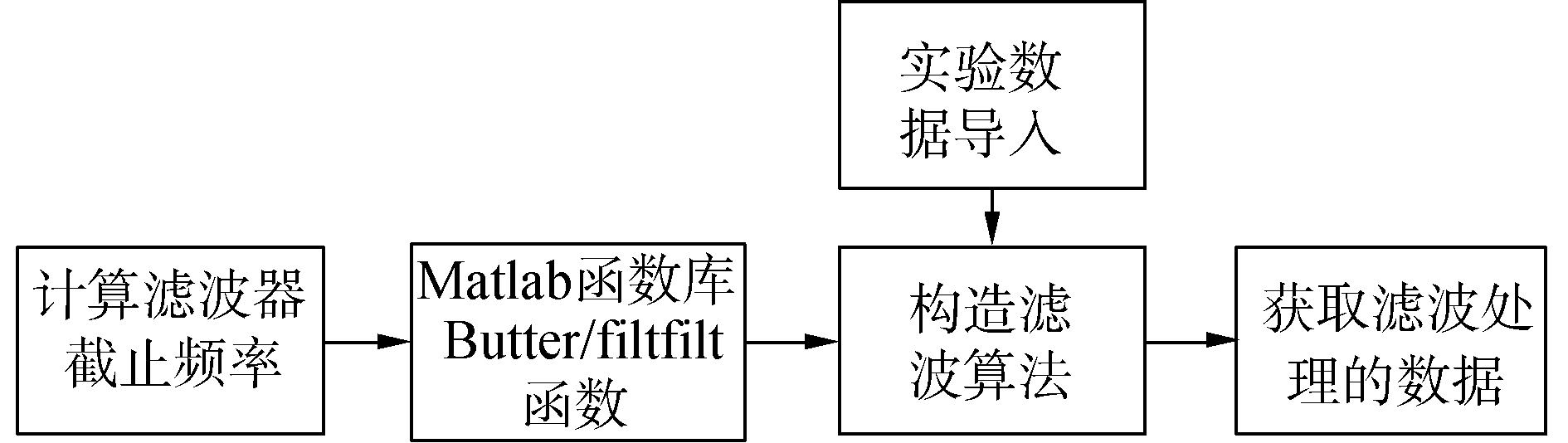Download: 图 4 滤波算法流程 Fig. 4 Flow chart of filtering algorithm

 ${\hat \chi _{{\rm{OLS}}}} = {\arg _{\rm{p}}}\min {\left\| {\mathit{\boldsymbol{\tau }} - D\mathit{\boldsymbol{\hat \chi }}} \right\|^2}$ (14)表 1 动力学参数辨识结果 Table 1 Identification results of dynamic parameters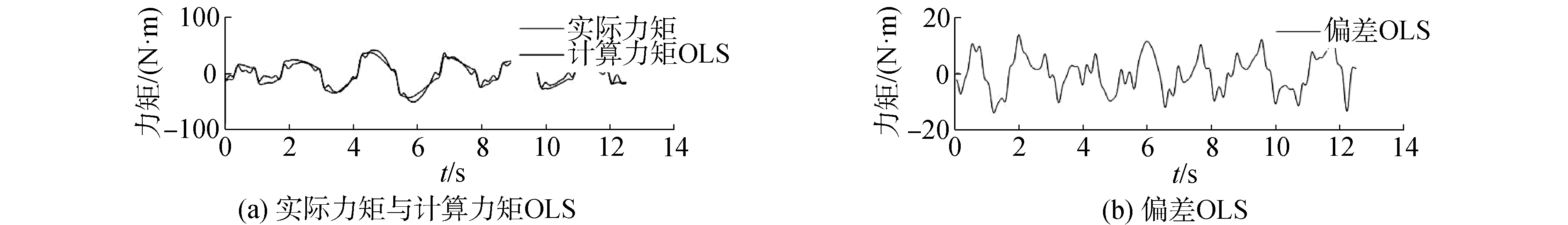Download: 图 5 动力学参数验证 Fig. 5 Dynamic parameter validation表 2 参数辨识结果 Table 2 Parameter identification results表 3 刚度系数辨识结果 Table 3 Results of stiffness factor identification
2 柔体动力学模型前馈补偿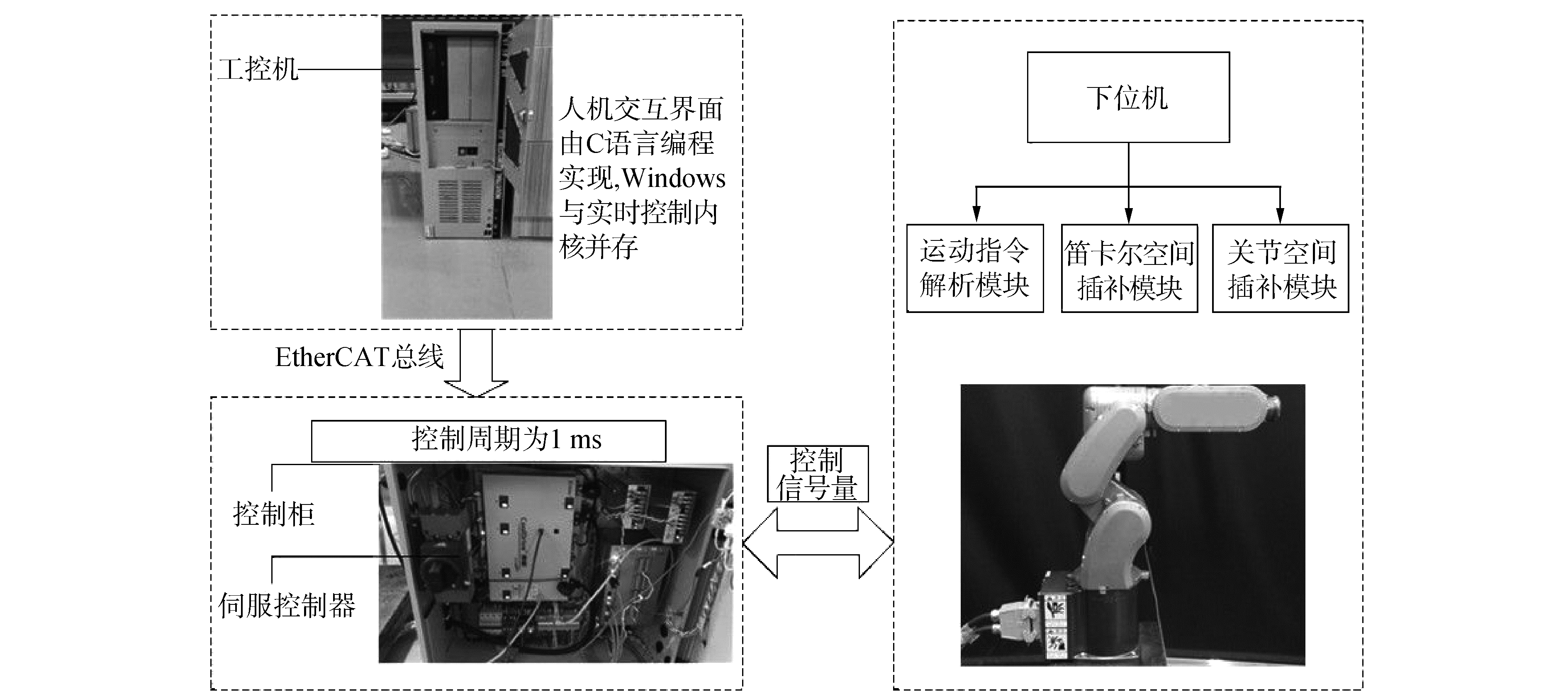Download: 图 6 机器人控制系统 Fig. 6 Robot control system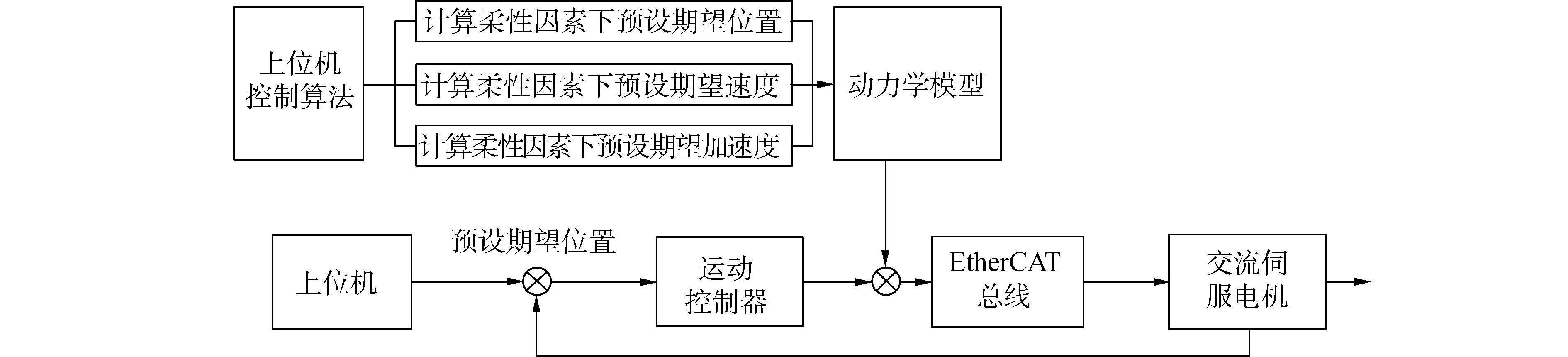Download: 图 7 基于柔体动力学模型的力矩前馈控制律 Fig. 7 Torque feedforward control law based on flexible body dynamics model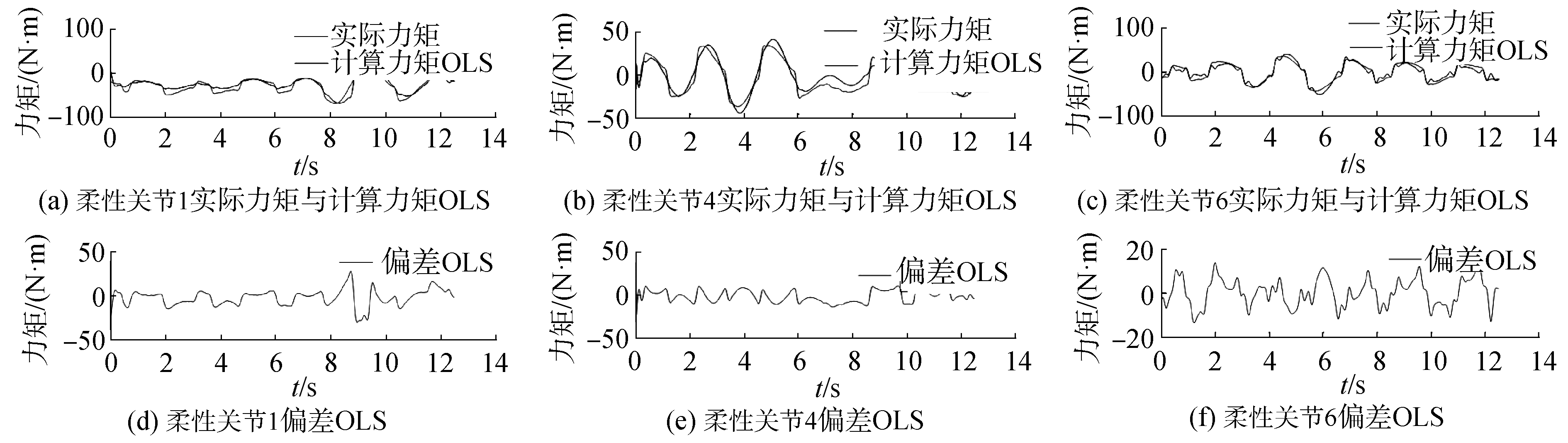Download: 图 8 柔性各个关节前馈力矩补偿验证 Fig. 8 Verification diagram of feedforward torque compensation for flexible joints

3 机器人动力学性能测试与实验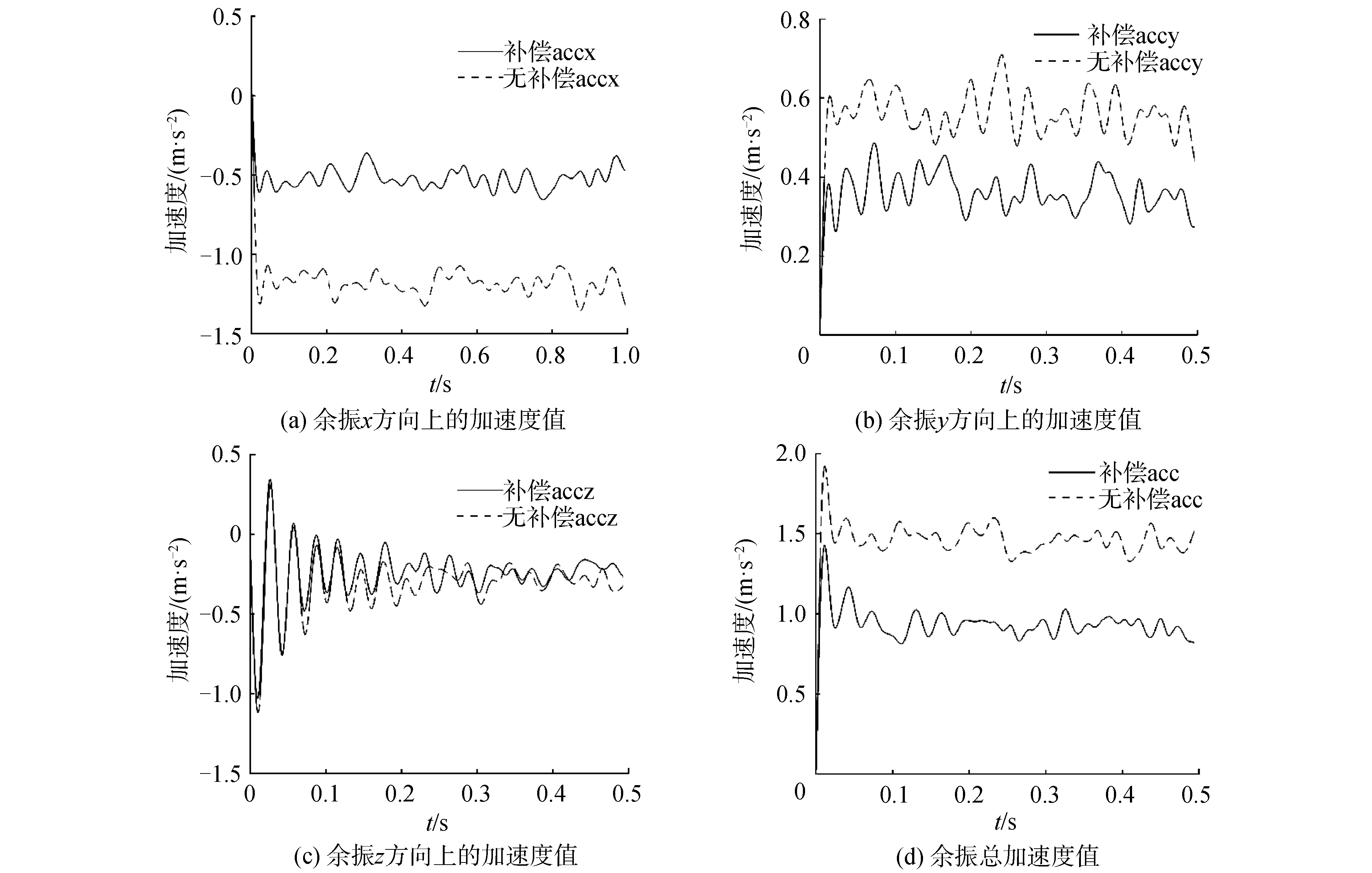Download: 图 9 速度段2 500 mm/s运动停止后末端余振加速度分析 Fig. 9 Acceleration analysis of end residual vibration after velocity 2 500 mm/s stop motion表 4 运动停止后末端余振加速度分析表 Table 4 The analysis table of the acceleration of the end residual vibration after the stop of motion

4 结论

1) 优化机器人的控制算法从而达到改善机器人动态特性的目的。根据机器人动力学特性进行机器人运动过程中的动态补偿，通过内控制回路使得机器人更易控制。

2) 将3 kg垂直六自由度工业机器人系统化为一个解耦的线性定常系统，柔体动力学模型的机器人柔性力矩前馈作为控制手段，即通过建立机器人柔性关节的动力学模型，辨识柔性关节的扭转刚度参数以及最小惯性参数，获取更为准确的柔性因素下的预设位置、速度、加速度信息。

3) 计算出柔性关节下所需的力矩值，最终实现机器人的柔性控制，使得机器人末端振动加速度幅值下降60%，提高了机器人末端的定位精度，满足了工业生产上对机器人的动态性能的要求。实验验证了柔体动力学模型的机器人柔性力矩前馈补偿控制具有一定的工程应用价值。

  FENG Fei, LIU Yiwei, LIU Hong, et al. Design schemes and comparison research of the end-effector of large space manipulator[J]. Chinese journal of mechanical engineering, 2012, 25(4): 674-687. DOI:10.3901/CJME.2012.04.674 (0)  杨益波.柔性关节柔性臂杆机械臂动力学建模与振动抑制研究[D].哈尔滨: 哈尔滨工业大学, 2015. YANG Yibo. The dynamics modeling and vibration sup pression research off lexible joint-flex ible link manipulators[D]. Harbin: Harbin Institute of Technology, 2015. (0)  ZHU Yaguang, JIN Bo, LI Wei. Leg compliance control of a hexapod robot based on improved adaptive control in different environments[J]. Journal of Central South University, 2015, 22(3): 904-913. DOI:10.1007/s11771-015-2600-0 (0)  MAKAROV M, GROSSARD M, RODRÍGUEZ-AYERBE P, et al. Modeling and preview H∞ control design for motion control of elastic-joint robots with uncertainties[J]. IEEE transactions on industrial electronics, 2016, 63(10): 6429-6438. DOI:10.1109/TIE.2016.2583406 (0)  AHMAD M A, MOHAMED Z, ISMAIL Z H. Experimental investigation of feedforward control schemes of a flexible robot manipulator system[J]. Elektrika:journal of electrical engineering, 2008, 10(2): 28-35. (0)  PAUL R P. Robot manipulators:mathematics, programming, and control:the computer control of robot manipulators[M]. London: The MIT Press, 1981: 164-172. (0)  OTT C. Cartesian impedance control of redundant and flexible-joint robots[M]. Berlin Heidelberg: Springer, 2008. (0)  CHAN M L. Controller synthesis and vibration suppression techniques for industrial robotic manipulators with joint flexibilities[D]. Berkeley: University of California, 2013. (0)  PHAM M T, GAUTIER M, POIGNET P. Identification of joint stiffness with bandpass filtering[C]//Proceedings of 2001 IEEE International Conference on Robotics and Automation. Seoul, South Korea, 2001: 2867-2872. (0)  DING Li, WU Hongtao, YAO Yu, et al. Dynamic model identification for 6-DOF industrial robots[J]. Journal of robotics, 2015, 2015: 471478. (0)  CORKE P. Robotics, vision and control[M]. Berlin: Springer, 2011. (0)  SOUSA C D, RUI C. Physical feasibility of robot base inertial parameter identification:A linear matrix inequality approach[J]. The international journal of robotics research, 2014, 33(6): 931-944. DOI:10.1177/0278364913514870 (0)